# Molecular Spectra

Also found in: Dictionary, Thesaurus.
The following article is from The Great Soviet Encyclopedia (1979). It might be outdated or ideologically biased.

## Molecular Spectra

optical emission and absorption spectra, as well as Raman scattering spectra, that belong to free or weakly bound molecules. Molecular spectra have a complex structure. Typical molecular spectra are band spectra, which are observed in emission, absorption, and Raman scattering in the form of the aggregate of more or less narrow bands in the ultra-violet, visible, and near-infrared regions and break down into a set of closely positioned lines when the spectral instruments used have sufficient resolving power. The specific structure of molecular spectra differs for different molecules and, generally speaking, grows more complex as the number of atoms in the molecule increases. For extremely complex molecules the visible and ultraviolet spectra consist of a few broad continuous bands; the spectra of such molecules are similar.

Molecular spectra arise during quantum transitions between the energy levels ℰ’ and ℰ” of molecules according to the relation

(1) hν = ℰ’ - ℰ”

where is the energy of the emitted or absorbed photon of frequency ν (h is Planck’s constant). During Raman scattering, is equal to the energy difference between the incident and scattered photons. Molecular spectra are much more complex than atomic line spectra because of the greater complexity of internal motion in molecules. In addition to the motion of electrons with respect to two or more nuclei, vibrational motion of the nuclei (together with the inner electrons that surround them) about equilibrium positions and rotational motion of the molecule as a whole take place in molecules. Three types of energy levels and three types of spectra—electronic, vibrational, and rotational—correspond to these three types of motion.

According to quantum mechanics, the energy of all types of motion in a molecule can assume only discrete values—that is, the energy is quantized. The total energy S> of a molecule may be represented approximately as the sum of the quantized values of the energy of all three types of its motion:

(2) ℰ = ℰe1 + ℰvib + ℰrot

In order of magnitude,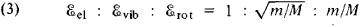where m is the mass of the electron, and the quantity M is of the order of the mass of atomic nuclei in a molecule—that is, m/M ∽ 10−3- 10−5. Consequently,

(4) ℰe1 ≫ ℰvib ≫ ℰrot

The quantity ℰei is usually of the order of a few electron volts (eV), or a few hundred kilojoules per mole (kJ/mol), ℰvibVib ∽ 10−2- 10−1 eV, and ℰrot ∽ 10−5-10−3 eV.

According to (4), the system of energy levels of a molecule is characterized by the aggregate of widely separated electron levels (the various values of ℰel when ℰvib = ℰrot = 0), much more closely situated vibrational levels (various values of ℰVib for a given ℰei and for ℰrot = 0), and still more closely situated rotational levels (various values of ℰrot for given ℰei and ℰVib). A diagram of the levels of a diatomic molecule is shown in Figure 1; for polyatomic molecules the system of levels is still more complex.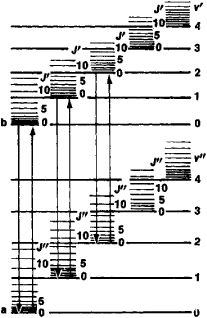Figure 1. Energy levels of a diatomic molecule: (a) and (b) electron levels,( v’) and ( v”) quantum numbers of the vibration levels,( J’) and( J”) quantum numbers of the rotational levels

The electron energy levels ei in (2) and in the diagram in Figure I correspond to the equilibrium configurations of the molecule (in the case of a diatomic molecule characterized by the equilibrium values ro of the internuclear distance r). A certain equilibrium configuration and a certain value of ℰei correspond to each electron state. The lowest value corresponds to the ground energy level.

The set of the electron states of a molecule is determined by the properties of its electron shell. In principle the values of ℰei may be calculated by the methods of quantum chemistry, but this task can be carried out only by approximate methods and for comparatively simple molecules. The most important information regarding the electron levels of a molecule (the position and characteristics of the electron energy levels), which is determined by its chemical structure, can be obtained by studying its molecular spectrum.

The value of the quantum number S, which characterizes the absolute value of the total spin moment of all electrons in the molecule, is an extremely important characteristic of a given electron energy level. Chemically stable molecules usually have an even number of electrons, and for them S = 0,1, 2, . . . (the value S = 0 is typical of the ground electron level, whereas 5 — 0 and S = 1 are typical of excited levels). Levels with S = 0 are called singlets, and those with S= 1 are called triplets (since the interaction in the molecule leads to splitting into K = 2S + 1 = 3 sublevels). Free radicals usually have an odd number of electrons; for them S = 1/2, 3/2,. . ., and the value 5=1/2 (doublet levels that split into K = 2 sublevels) is typical of both the ground and excited levels.

For molecules whose equilibrium configurations are symmetrical, the electron levels may be classified in another way. In the case of diatomic and linear triatomic molecules that have an axis of symmetry (of infinite order) that passes through the nuclei of all atoms (see Figure 2,b), the electron levels are characterized by values of the quantum number λ, which defines the absolute value of the projection of the total orbital moment of all electrons onto the axis of the molecule. Levels with λ = 0, 1, 2, . . . are correspondingly designated as Σ, Π, Δ , . . . , and the quantity K: is indicated by a superscript on the left (for example, 3Σ.2Π … ) For molecules that have a center of symmetry, such as CO2 and C6H6 (see Figure 2, b and c), all electron levels are divided into even and odd levels, which are designated by the indices g and u (depending on whether the wave function retains its sign or changes it upon reversal at the center of symmetry). The vibrational energy levels (the values of ℰVib) can be found by quantizing the vibrational motion, which may be considered to be approximately harmonic. In the simplest case of a diatomic molecule (one vibrational degree of freedom, corresponding to a change in the internuclear distance r) it is considered as a harmonic oscillator; quantization gives the equidistant energy levels:

(5) ℰvib = e (ν +1/2)

where νe is the fundamental frequency of the harmonic vibrations of the molecule, and ν is the vibrational quantum number, which assumes the values 0, 1, 2,. … The vibrational levels for two electron states are illustrated in Figure 1.

A total of f “normal oscillations,” with frequences νi (i=1, 2, 3, …, f) and a complex system of vibrational levels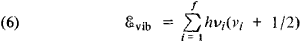where νi= 0, 1, 2, … are the corresponding vibrational quantum numbers, can be obtained for every electron state of a polyatomic molecule consisting of N atoms ( N ≥ 3) with f vibrational degrees of freedom (f = 3 N — 5 and f = 3 N — 6 for linear and nonlinear molecules, respectively). The set of frequencies of normal vibrations in the ground electron state is a very important characteristic of a molecule and depends on its chemical structure. All or part of the atoms of a molecule participate in a certain normal vibration; in the process, the atoms perform harmonic vibrations with the same frequency νi but with different amplitudes that determine the shape of the vibration. Normal vibrations are divided according to shape into stretching vibrations (in which the lengths of the bond lines change) and deformational vibrations (in which the angles between chemical bonds—the valence angles—change). The number of vibration frequencies is 2 for molecules of low symmetry (molecules that do not have axes of symmetry of an order greater than 2), and all vibrations are nondegenerate; for more symmetrical molecules there are doubly or triply degenerate oscillations (pairs and triplets of frequency-coincident vibrations). For example, for the nonlinear triatomic molecule H2O (Figure 2,a) f= 3, and three nondegenerate oscillations (two stretching vibrations and one deformational vibration) are possible. The more highly symmetrical linear triatomic molecule CO2 (Figure 2,b) has f = A —two nondegenerate (stretching) vibrations and one doubly degenerate (deformational) vibration. For the plane high-symmetry molecule C6H6 (Figure 2,c) we obtain f = 30—ten nondegenerate and ten doubly degenerate vibrations; of these, 14 take place in the plane of the molecule (eight stretching and six deformational vibrations) and six nonplanar deformational vibrations take place perpendicular to the plane. The still more highly symmetric tetrahedral molecule CH4 (Figure 2,d) has f = 9—one nondegenerate (stretching) vibration, one doubly degenerate (deformational) vibration, and two triply degenerate vibrations (one stretching and one deformational).

The rotational energy levels may be found by quantizing the rotational motion of the molecule if it is considered as a solid with well-defined moments of inertia. In the simplest case, a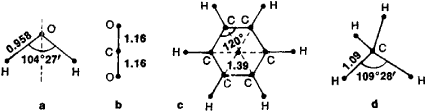Figure 2. Equilibrium configurations of molecules: (a) H2O, (b) CO2, (c) C6H6, (d) CH4. The numbers indicate the bond lengths (in angstroms) and the values of the valence angles.

diatomic or linear multiatomic molecule, its energy of rotation is

rot = 1/2( M2/1)

where I is the molecule’s moment of inertia with respect to the axis perpendicular to the axis of the molecule, and M is the angular momentum. According to the rules of quantization,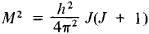where the rotational quantum number J = 0, 1, 2, … and, consequently, the following is obtained for ℰrot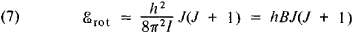where the rotational constant B = h/8π2I defines the scale of the distances between energy levels, which decreases as the masses of the nuclei and the internuclear distances increase. The rotational levels for each electronic-vibrational state are illustrated in Figure 1.

Various types of molecular spectra arise during different types of transitions between the energy levels of molecules. According to (1) and (2),

(8) Δℰ = ℰ’ - ℰ” = Δℰcl + Δℰvib + Δℰrot

where changes in Δℰei, Δℰvib, and Δℰrot (the electronic, vibrational, and rotational energies, respectively) satisfy the condition

(9) Δℰel ≫ Δℰvib ≫ Δℰrot

The distances between levels are of the same order as the energies ℰei, ℰvib, and ℰrot themselves, which satisfy condition (4).

When Δℰel ≠ 0, electron molecular spectra that can be seen in the visible and ultraviolet (UV) regions are produced. When Δℰel ≠ 0, usually Δℰvib ≠ 0 and Δℰrot ≠0; different vibrational bands correspond to different Δℰvib for a given Δℰei, and the different rotational lines into which the given band breaks down correspond to different Δℰrot for given Δℰei and Δℰvib,. The characteristic band structure is obtained. The aggregate of bands with a given ASei (which corresponds to a purely electron transition of frequency vei = Δℰei/h) is called a system of bands; the individual bands have different intensities that depend on the relative probabilities of the transitions, which can be calculated approximately by quantum-mechanical methods. For complex molecules, the bands of one system that correspond to a given electron transition usually merge into one broad continuous band, and several such broad bands may be superimposed on one another. Characteristic discrete electron spectra are observed in frozen solutions of organic compounds. Electron (or, more accurately, electron-vibrational-rotational) spectra are studied experimentally with spectrographs and spectrometers that use glass optics (for the visible region) and quartz optics (for the UV region), in which prisms or diffraction gratings are used to split the light into a spectrum.

When Δℰei = 0 and Δℰvib ≠0, vibrational molecular spectra are obtained that can be observed in the near infrared region (up to a few microns) and the central infrared region (up to a few dozen microns), usually in absorption but also in Raman scattering of light. When Δℰrot ≠ 0 and for a given ℰvib, a vibrational band that breaks down into individual rotational lines is usually obtained. Bands that correspond to Δνi = ν’i - ν”i =I when Δνk = ν’k - ν” k= 0 where k ≠ i

These selection rules, which forbid other transitions, are strictly satisfied for purely harmonic vibrations. For anharmonic vibrations, bands for which Δν > 1 (overtones) appear. Their intensity is usually small and decreases as Δν increases.

Vibrational (or, more accurately, vibrational-rotational) spectra are studied experimentally in absorption in the infrared region by means of infrared spectrometers with prisms that are transparent to infrared radiation or with diffraction gratings, and also by means of Fourier spectrometers, and, in Raman scattering, by means of high-transmission spectrographs (for the visible region) using laser excitation.

When Δℰei = 0 and Δℰvib = 0, purely rotational molecular spectra that consist of individual lines are produced. They are observed in absorption in the remote infrared region (hundreds of microns) and especially in the microwave region, and also in Raman spectra. For diatomic and linear polyatomic molecules (and also for sufficiently symmetric nonlinear polyatomic molecules), the lines are equidistant (in the frequency scale) at intervals of Δν = 2B in absorption spectra and Δν = 4 B in Raman spectra.

Purely rotational spectra are studied in absorption in the remote infrared region by means of infrared spectrometers with special diffraction gratings (echelette gratings) and Fourier spectrometers, whereas microwave (SHF) spectrometers are used in the microwave region and high-transmission spectrographs are used in Raman scattering.

Methods of molecular spectroscopy, which are based on the study of molecular spectra, make it possible to solve various problems of chemistry, biology, and other sciences (for example, to determine the composition of petroleum products and polymers). In chemistry, molecular structure is studied on the basis of molecular spectra. Electron molecular spectra make it possible to obtain information on the electron shells of molecules, to determine excited levels and their characteristics, and to find the dissociation energies of molecules (from the convergence of the vibrational levels of the molecule toward the dissociation boundaries). The study of vibrational molecular spectra makes it possible to find the characteristic frequencies of vibrations that correspond to certain types of chemical bonds in a molecule (for example, simple double and triple C—C bonds and C—H, N— H, and O—H bonds for organic molecules) of various groups of atoms (such as CH2, CH3, and NH2), to determine the three-dimensional structure of molecules, and to distinguish between cis- and trans- isomers. Both infrared absorption spectra and Raman scattering spectra are used for this purpose. The infrared absorption spectrum method has come into particularly wide use as one of the most effective optical methods of studying molecular structure. It provides the most complete information when combined with the study of Raman scattering spectra. The study of rotational molecular spectra and the rotational structure of electron and vibrational spectra makes it possible to find the parameters of the equilibrium configuration of a molecule (the bond length and valence angles) with high precision (for simpler molecules such as H2O) from experimentally determined values of the moments of inertia of molecules, which are obtained from the values of the rotational constants (see ). The spectra of isotopic molecules (in particular, molecules in which hydrogen is replaced by deuterium), which have identical parameters of their equilibrium configurations but different moments of inertia, are studied to increase the number of parameters that can be determined.

Let us examine the benzene molecule, CeHe, as an example of the use of molecular spectra to determine the chemical structure of molecules. The study of its molecular spectrum confirms the correctness of the model of a planar molecule in which all six C—C bonds in the benzene ring are equivalent and form a regular hexagon (Figure 2,c), which has a sixth-order axis of symmetry that passes through the center of symmetry of the molecule perpendicular to its plane. The molecular electron absorption spectrum of C6H6 consists of several systems of bands that correspond to transitions from the ground, even singlet level to excited odd levels, the first of which is a triplet and the higher levels are singlets (Figure 3). The system of bands is most intensive in the region of 1840 angstroms (Å), where ℰ5 — ℰ1 = 7.0 eV, and the system of bands in the region of 3400 Å (ℰ2 — ℰ1 = 3.8 eV), which corresponds to a singlet-triplet transition prohibited by the approximate selection rules for total spin, is the weakest. The transitions correspond to the excitation of π-electrons, which are delocalized over the entire benzene ring.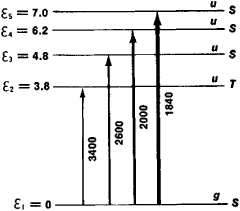Figure 3. Electron levels and transitions for the benzene molecule (the energy of the levels is given in electron volts): (S) singlet levels, (7) triplet level. The parity of the levels is indicated by the letters g and u. Approximate wavelength regions (in angstroms) are indicated for systems of absorption bands; more intensive systems of bands are indicated by heavier arrows.

The pattern of the levels obtained from the electron molecular spectra (Figure 3) is in agreement with approximate quantum-mechanical calculations. The vibrational molecular spectra of C6H6 correspond to the presence in the molecule of a center of symmetry: vibration frequencies that are manifested in the infrared absorption spectrum (active frequencies) are absent (inactive) in the Raman scattering spectrum, and vice versa (called alternative prohibition). Of the 20 normal oscillations of C6H6, four are active in the infrared absorption spectrum and seven are active in the Raman scattering spectrum; the other 11 are inactive in both. The values of the measured frequencies (in cm−1) are 673, 1038, 1486, and 3080 in the infrared absorption spectrum and 607, 850, 992, 1178, 1596, 3047, and 3062 in the Raman scattering spectrum. The frequencies 673 and 850 correspond to nonplanar vibrations, and all other frequencies correspond to planar vibrations. The frequencies 992 (which corresponds to stretching vibration of the C—C bonds, which consists in periodic compression and expansion of the benzene ring), 3062 and 3080 (which correspond to stretching vibrations of the C—H bonds), and 607 (which corresponds to deformational vibration of the benzene ring) are especially characteristic of planar vibrations. The observed vibrational spectra of C6H6 (and the analogous vibrational spectra of C6D6) are in very good agreement with the theoretical calculations that made it possible completely to interpret the spectra and to find the forms of all normal vibrations.

The structure of various classes of organic and inorganic molecules, including extremely complex molecules such as those of polymers, may be determined in the same manner by using the molecular spectra.

### REFERENCES

Kondrat’ev, V. N. Struktura atomov i molekul, 2nd ed. Moscow, 1959.
El’iashevich, M. A. Atomnaia i molekuliarnaia spektroskopiia. Moscow, 1962.
Herzberg, G.Spektry i stroenie dvukhatomnykh molekul. Moscow, 1949. (Translated from English.)
Herzberg, G. KolebateVnye i vrashchateVnye spektry mnogoatomnykh molekul. Moscow, 1949. (Translated from English.)
Herzberg, G. Elektronnye spektry i stroenie mnogoatomnykh molekul. Moscow, 1969. (Translated from English.)
Primenenie spektroskopii v khimii. Edited by V. West. Moscow, 1959. (Translated from English.)

M. A. EL’IASHEVICH

The Great Soviet Encyclopedia, 3rd Edition (1970-1979). © 2010 The Gale Group, Inc. All rights reserved.
Site: Follow: Share:
Open / Close